# Fraction calculator

The calculator performs basic and advanced operations with fractions, expressions with fractions combined with integers, decimals, and mixed numbers. It also shows detailed step-by-step information about the fraction calculation procedure. Solve problems with two, three, or more fractions and numbers in one expression.

## Result:

### 10/9 = 10/9 = 1 1/9 ≅ 1.1111111

Spelled result in words is ten ninths (or one and one ninth).

#### Rules for expressions with fractions:

Fractions - use the slash “/” between the numerator and denominator, i.e., for five-hundredths, enter 5/100. If you are using mixed numbers, be sure to leave a single space between the whole and fraction part.
The slash separates the numerator (number above a fraction line) and denominator (number below).

Mixed numerals (mixed fractions or mixed numbers) write as non-zero integer separated by one space and fraction i.e., 1 2/3 (having the same sign). An example of a negative mixed fraction: -5 1/2.
Because slash is both signs for fraction line and division, we recommended use colon (:) as the operator of division fractions i.e., 1/2 : 3.

Decimals (decimal numbers) enter with a decimal point . and they are automatically converted to fractions - i.e. 1.45.

The colon : and slash / is the symbol of division. Can be used to divide mixed numbers 1 2/3 : 4 3/8 or can be used for write complex fractions i.e. 1/2 : 1/3.
An asterisk * or × is the symbol for multiplication.
Plus + is addition, minus sign - is subtraction and ()[] is mathematical parentheses.
The exponentiation/power symbol is ^ - for example: (7/8-4/5)^2 = (7/8-4/5)2

#### Examples:

subtracting fractions: 2/3 - 1/2
multiplying fractions: 7/8 * 3/9
dividing Fractions: 1/2 : 3/4
exponentiation of fraction: 3/5^3
fractional exponents: 16 ^ 1/2
adding fractions and mixed numbers: 8/5 + 6 2/7
dividing integer and fraction: 5 ÷ 1/2
complex fractions: 5/8 : 2 2/3
decimal to fraction: 0.625
Fraction to Decimal: 1/4
Fraction to Percent: 1/8 %
comparing fractions: 1/4 2/3
multiplying a fraction by a whole number: 6 * 3/4
square root of a fraction: sqrt(1/16)
reducing or simplifying the fraction (simplification) - dividing the numerator and denominator of a fraction by the same non-zero number - equivalent fraction: 4/22
expression with brackets: 1/3 * (1/2 - 3 3/8)
compound fraction: 3/4 of 5/7
fractions multiple: 2/3 of 3/5
divide to find the quotient: 3/5 ÷ 2/3

The calculator follows well-known rules for order of operations. The most common mnemonics for remembering this order of operations are:
PEMDAS - Parentheses, Exponents, Multiplication, Division, Addition, Subtraction.
BEDMAS - Brackets, Exponents, Division, Multiplication, Addition, Subtraction
BODMAS - Brackets, Of or Order, Division, Multiplication, Addition, Subtraction.
GEMDAS - Grouping Symbols - brackets (){}, Exponents, Multiplication, Division, Addition, Subtraction.
Be careful, always do multiplication and division before addition and subtraction. Some operators (+ and -) and (* and /) has the same priority and then must evaluate from left to right.

## Fractions in word problems:

• Larry 2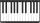Larry spends half of his workday teaching piano lessons. If he sees 6 students, each for the same amount of time, what fraction of his workday is spent with each student?
• Unknown numberI think the number - its sixth is 3 smaller than its third.
• Original numberJoe divided a number by 2 1/4 to get 2/3. What was the original number?
• I have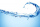I have 1/5 of an ounce of water left. I separate the water equally into two cups. How many ounces of water are in each cup?
• Cakes1/3 poppy cake, 1/3 apple, 15 pieces of cheese. How many are totally cakes?
• Two divided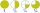Two divided by nine-tenths.
• Farmer PeterFarmer Peter paints 12 chicken coop. He started painting this day morning. Now he only has 1/4 of the chicken coop left to paint this afternoon. How many chicken coops did farmer Peter paint this morning?
• Brick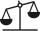Isosceles scale has on one side all brick and second weight 1 kg and 1/4 of brick. The balance is in equilibrium. What is the weight of a brick?
• Athletic raceBefore a race, you start 4 5/8 feet behind your friend. At the halfway point, you are 3 2/3 feet ahead of your friend. What is the change in distance between you and your friend from the beginning of the race?
• Sales taxA sales tax on a Php 10,800 appliance is Php.1,620. What is the rate of sales tax?
• Wood 11Father has 12 1/5 meters long wood. Then I cut the wood into two pieces. One part is 7 3/5 meters long. Calculate the length of the other wood?
• A manA man spends 5/9 of his money on rent, and 5/16 of the remainder on electricity. If the final balance remaining is 550 find how much was spent on rent
• Diamonds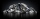If simva had 8 diamond and cuts them each into 1/3 pieces, then how many pieces does she have?